Module 21 - Sequences and Series

Introduction | Lesson 1 | Lesson 2 | Lesson 3 | Self-Test

Lesson 21.1: Sequences

In this lesson you will investigate sequences by using the TI-89's features. You will also explore when a sequence converges by viewing the graph of a sequence and the table of associated values.

Defining a Sequence

A sequence is a function whose domain is restricted to the set of positive integers or, in some cases, the set of nonnegative integers. The numerical representation for a sequence is a list or table of values and the graphical representation is a set of discrete points. There are several TI-89 features that are useful in creating these representations.

Using the Sequence Command

The sequence command can be used to create a list of a finite number of terms from a sequence.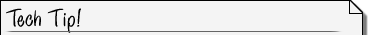The Sequence Command The sequence command, seq(, is found in the MATH List menu and in the Catalog. The syntax for this command is seq(expression,var,low,high, STEP) "Expression" is evaluated for various values of "var" with the first value being "low" and the last value being "high." The 5th parameter "STEP" is optional and sets the step size for incrementing the index variable "var" starting at "low." If no "STEP" is entered the step size defaults to 1. If low = 6 and high = 10, the TI-89 will compute the 6th term through the 10th term of the sequence given by expression and display those terms in a list.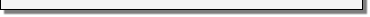Finding the Terms of a Sequence

Find the first ten terms of the sequence defined by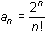, where an represents the value of the nth term of the sequence. Recall that the symbol "!" represents the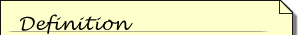The factorial of a whole number is the product of the series of consecutive whole numbers that begin with 1 and end with the number: 6! = 1x2x3x4x5x6. 0! is defined to be 1.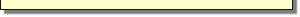factorial of the preceding number.

• Perform NewProb
• Open the MATH menu by pressing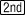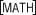•is above• Highlight 3:List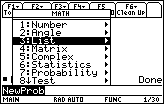• Open the List menu by pressingor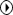The first option in this menu is 1:seq(.

• Paste this command to the Home Screen by pressing• Complete the command seq(2^n/n!, n, 1, 10)
• Press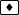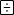for the factorial symbol "!," which can also be found in the Catalog.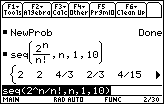The command generates a list containing the first 10 terms in the sequence. Move the cursor into the History Area and scroll to the right to see the hidden part of the list.

21.1.1 Describe the behavior of list generated by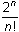as n gets large.

21.1.2 Take the limit of the sequence as n approachesby entering limit(2^n/n!, n,).

Defining Convergence

Because the limit of the expression that defines the sequence exists as n approaches infinity, the sequence is said to converge to the value of the limit. The sequence defined byconverges to 0 as found in Question 21.1.2.

If the limit grows without bound or the values jump around or oscillate and do not approach a single value, the sequence is divergent. A sequence may oscillate and converge if the oscillations become small and the values approach a single value.

Graphing Sequences

A graph of the sequence illustrates convergence.Sequence Graph Mode Variables The independent variable for Sequence Graph mode is n and the expressions that define sequences in the Y= Editor are u1, u2, etc.• Select Sequence Graphing mode by pressing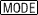to open the Graph mode dialog box and highlighting 4:Sequence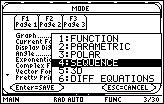• Save the setting by pressingtwice
• Enter the expression 2^n/n! in the Y= Editor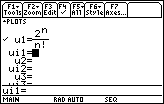• Enter the Window values shown below
• A description of the new parameters is given in the first column.

 Beginning value of n nmin = 1 xmin = 0 ymin = -1 Ending value of n nmax = 10 xmax = 11 ymax = 3 Where the plot starts plotStrt = 1 xscl = 1 yscl = 1 The increase in n from one point to the next plotStep = 1

• Display the graph of the sequence by pressing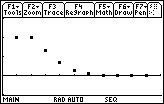TRACE the terms of the sequence.

21.1.3 What feature of the graph indicates that the sequence converges?

Creating a Table of Values

A sequence that is defined in the Y= Editor can be displayed in a table.

• Open the Table Setup dialog box by pressingand set both tblStart and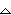tbl to 1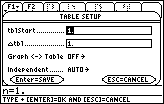• Be sure to pressin order to save the settings when you exit the dialog box
• Use the cursor movement keys to scroll down the table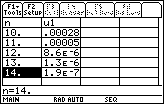As you scroll down the table the values of u1 get smaller and smaller, which is numerical evidence that the sequence converges to zero.

21.1.4 Does the sequence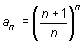converge? Provide graphical, numerical and analytical evidence for your conclusion.